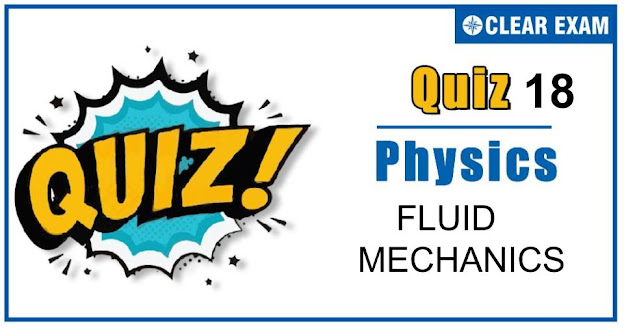## FLUID MECHANICS QUIZ-18

As per analysis for previous years, it has been observed that students preparing for NEET find Physics out of all the sections to be complex to handle and the majority of them are not able to comprehend the reason behind it. This problem arises especially because these aspirants appearing for the examination are more inclined to have a keen interest in Biology due to their medical background. Furthermore, sections such as Physics are dominantly based on theories, laws, numerical in comparison to a section of Biology which is more of fact-based, life sciences, and includes substantial explanations. By using the table given below, you easily and directly access to the topics and respective links of MCQs. Moreover, to make learning smooth and efficient, all the questions come with their supportive solutions to make utilization of time even more productive. Students will be covered for all their studies as the topics are available from basics to even the most advanced. .

Q1. The onset of turbulence in a liquid is determined by
•  Pascal’s law
•  Magnus effect
•  Reynold’s number
•  Bernoulli’s principle
Solution
(i) FBD of the cube with respect to the container B=(m/σ)ρg_eff=m(ρ/σ) √(a2+g2 ) tan⁡θ=a/g sin⁡θ=a/√(a2+g2 );cos⁡θ=g/√(a2+g2 ) Let acceleration of the cube w.r.t. container in horizontal direction in ax' mg+B sin⁡θ-mg/2=max ax=g/2+ρ/σ a=ax⇒g/2+ρ/σ∙g/2=ax Cart: (T+B) sin⁡θ'mg (i) (T+B) cos⁡θ'=mg (ii) tan⁡θ'=a/g (iii) Hence, tan⁡θ'/tan⁡θ =1/1=θ'/θ=1:1

Q2.An object weighs m_1 in a liquid of density d1 and that in liquid of density d2 is m2. The density d of the object is
•  d=(m2 d2-m1-d1)/(m2-m1)
•  d=(m1d1-m2-d2)/(m2-m1 )
•  d=(m2 d1-m1-d2)/(m1-m2 )
•  d=(m1 d2-m2-d1)/(m1-m2 )
Solution
V(d-d1 )g=m1 g V(d-d2 )g=m2 g (d-d1)/(d-d2 )=m1/m2 ∴ d=(m1 d2-m2 d1)/(m1-m2 )

Q3.  Water flows steadily through a horizontal pipe of variable cross-section. If the pressure of water is p at a point where flow speed is v, the pressure at another point where the flow of speed is 2v, is (take density of water as ρ)
•   p-(3ρv2)/2
•  p-〖ρv〗2/2
•  p-(3〖ρv〗2)/4
•  p-ρv2
Solution
From Bernoulli’s equation, the sum of all forms of energy in a fluid flowing along an enclosed path (a streamline) is the same at any two points in the path. Therefore, p+1/2 ρv12=p'+1/2 ρv22 Given,v2=2v, v1=v ∴ p+1/2 ρv2=p'+1/2 ρ(2v)2 ⟹ p^'= p-3/2 ρv2

Q4. A cylindrical vessel is filled with equal amounts of weight of mercury on water. The overall height of the two layers is 29.2 cm, specific gravity of mercury is 13.6. Then the pressure of the liquid at the bottom of the vessel is
•  29.2 cm of water
•  29.2 /13.6 cm of mercury
•  4 cm of mercury
•  15.6 cm of mercury
Solution
Let A be the area of cross-section of the cylindrical vessel and x cm be the height of mercury in vessel. The height of water in the vessel =(29.2×x) cm As per question Ax×13.6=(29.2-x)×1 or x=2 cm ∴ Height of water column =(29.2-2)=27.2 cm ∴ Pressure of the liquids at the bottom =27.2 cm of water column +2 cm of Hg column =27.2/13.6 of Hg column +2 cm of Hg column =4 cm of Hg column

Q5.When a glass capillary tube of radius 0.015 cm is dipped in water, the water rises to height of 15 cm within it. Assuming contact angle between water and glass to be 0°, the surface tension of water is [ρ_(water )=1000 kg m(-3),g=9.81 ms(-2)]
•  0.11Nm(-1)
•  0.7Nm(-1)
•  0.072 Nm(-1)
•  None of these
Solution
2πr×T cosθ=πr2 hρg ⟹ T=rhρg/2=0.11Nm(-1)

Q6. The amount of work done in blowing a soap bubble such that its diameter increases from d to D is (S= surface tension of solution)
•  π(D2-d2 )S
•  2π(D2-d2 )S
• 4π(D2-d2 )S
•  8π(D2-d2 )S
Solution
Change in surface area 2×4π[(D/2)2-(d/2)2]=2π(D2-d2) Work done = surface tension × change in area =S×2π(D2-d2)

Q7.A monometer connected to a closed tap reads 4.5×〖10〗5 pascal. When the tap is opened the reading of the monometer falls to 4×〖10〗^5 pascal. Then the velocity of flow of water is
•  7 ms(-1)
•  8 ms(-1)
•  9 ms(-1)
•  10 ms(-1)
Solution
(P1-P2 )/ρg=v2/2g⇒(4.5×〖10〗5-4×〖10〗5)/(〖10〗3×g)=v2/2g ∴v=10m/s

Q8.Soap bubbles can be formed floating in air by blowing soap solution in air, with the help of a glass tube but not water bubbles. It because
•  The excess pressure inside water bubble being more due to large surface tension
•  TThe excess pressure inside water bubble being less du large surface tension
•  The excess pressure inside water bubble being more due to large viscosity
•  The excess pressure inside water bubble being less due to less viscosity
Solution
The excess pressure inside water bubble being more due to large surface tension

Q9.A large open tank has two holes in its wall. One is a square hole of side a at a depth of x from the top and the other is a circular hole of radius r at a depth 4x from the top. When the tank is completely filled with water, the quantities of water flowing out per second from both holes are the same. Then r is equal to
•  2πa
•  A
•  a/2π
•  a/π
Solution
We have v2=ρgh a2 √ρgh=πr2 √ρgh×2 r=a/2π

Q10. If the atmospheric pressure is Pa, then the pressure P at depth h below the surface of a liquid of density ρ open to the atmosphere is
•  Pa-ρgh/2
•  Pa-ρgh
•  Pa
• Pa+ρgh
Solution
P=Pa+ρgh## Want to know more

Please fill in the details below:

## Latest NEET Articles\$type=three\$c=3\$author=hide\$comment=hide\$rm=hide\$date=hide\$snippet=hide

Name

ltr
item
BEST NEET COACHING CENTER | BEST IIT JEE COACHING INSTITUTE | BEST NEET, IIT JEE COACHING INSTITUTE: FLUID MECHANICS QUIZ-18
FLUID MECHANICS QUIZ-18